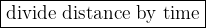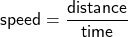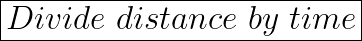## How is speed calculated? multiply velocity by displacement divide velocity by displacement multiply distance by time divide distance by time

Question

How is speed calculated? multiply velocity by displacement divide velocity by displacement multiply distance by time divide distance by time

in progress 0
3 months 2021-08-09T12:26:08+00:00 2 Answers 10 views 0Explanation:

Using the formula to calculate speed:We divide the distance by the time taken to get the average speed.